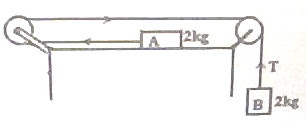## Pages

`“Life is like riding a bicycle.  To keep your balance you must keep moving.”–Albert Einstein`

## Friday, September 11, 2009

### AP Physics B & C – Two Multiple Choice Questions (for practice) on Newton’s Laws of Motion

"Only two things are infinite, the universe and human stupidity, and I'm not sure about the former."
Albert Einstein

Two practice questions (MCQ) on Newton’s laws of motion are given below with solution. Even though these are meant for AP Physics C aspirants, they should not be difficult for those who are preparing for AP Physics B Exam.(1) In the system shown in the adjoining figure, the horizontal surface on which block A is placed is smooth and the pulleys are light and frictionless. If g = 10 ms–2, the tension T in the string (which may be assumed to be weightless and inextensible) is
(a) 1 N
(b) 2N
(c) 5 N
The velocity of the iron block at the moment of hitting the nail is 6 ms–1.
(d) 10 N
(e) 20 N
Net force driving the system = Weight of B = mg = 2×10 = 20 N.
Acceleration (a) of the system is given by
a = Driving force /Total mass moved = 20/4 = 5 ms–2.
Tension, T = Weight of B moving down (as in a lift) = m(ga) = 2(10 – 5) =10 N.(2) An iron block of mass 8 kg falling vertically down hits a nail on a block of wood (fig.). The velocity of the iron block at the moment of hitting the nail is 6 ms–1. If the nail penetrates through 2 cm into the wooden block due to the impact, what is the force exerted by the iron block on the nail?
(a) 1600 N
(b) 2200 N
(c) 4800 N
(d) 7200 N
(e) 9600 N
The acceleration (in fact, retardation), a of the iron block during the impact is given by
0 = 62 + 2a×(0.02), from the equation v2 = u2 + 2as
This gives a = – 900 ms–2
The negative sign shows that the iron block is retarded.
The retarding force exerted by the nail on the iron block is of magnitude F given by
F = ma = 8×900 = 7200 N
The force exerted by the iron block on the nail is equal and opposite to that exerted by the nail on the iron block. The answer therefore is 7200 N.
[The above question can be modified as follows:
An iron block of mass 8 kg falling freely from rest under gravity from a height of 1.8 m hits a nail on a block of wood (fig.). If the nail penetrates through 2 cm into the wooden block due to the impact, what is the force exerted by the iron block on the nail? (g = 10 ms–2)
(a) 1600 N
(b) 2200 N
(c) 4800 N
(d) 7200 N
(e) 9600 N
The answer will be unchanged since the velocity at the moment of hitting the nail will be 6 ms–1 itself (v = √(2gh) = √(2×10×1.8) = √(36) = 6)].